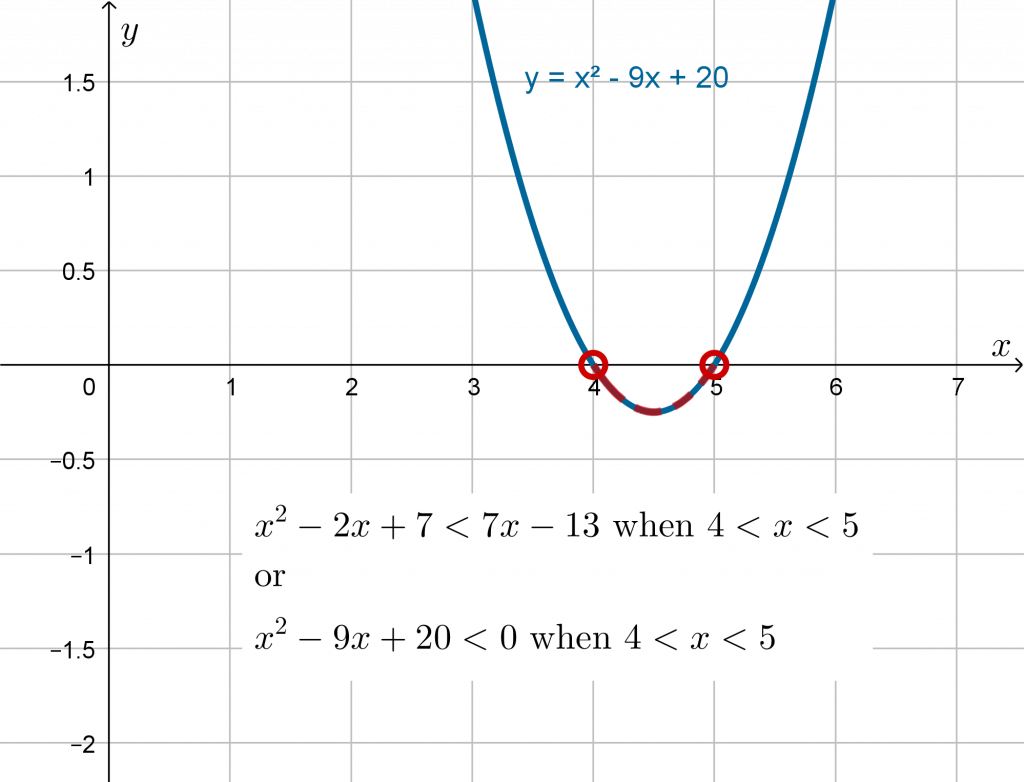# Introduction

An inequality is used when a range of values satisfies a condition.

Example 1 In many contexts it makes more sense to use an interval of values rather than a specific value. E.g. a nutritionist may recommend that a person aim consume between 1200 and 1800 mg sodium per day;  rather than exactly 1500 mg per day.

Example 2 We use an inequality to express each of the domain and range of a function:Note that an empty point us used for ‘less than’ or ‘greater than’ but not equal to; a solid point is used to represent ‘less than or equal to‘ or ‘greater than or equal to‘.

# Intervals on the number line

A single interval or inequality can be represented on a number line. Here are some examples:

# Solve Linear Inequalities

Example 3 An inequality such asbehaves in a very similar way to solving an equation. However instead of finding one single solution that satisfies the equation, we find the interval of solutions that satisfy the equation.Any value of, as long as it is less than or equal to 4, will satisfy this inequality.

Let’s try:It is true thatso this is correct.

Let’s try: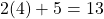It is true that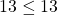so this is correct.

Let’s tryThis is not in our solution interval.. This is not less than or equal to 13, and so 4.1 is not a solution.

To understand this result visually, we graph(blue) and(green). Notice that theon the blue line is less than (below) theon the green line for allvalues up to.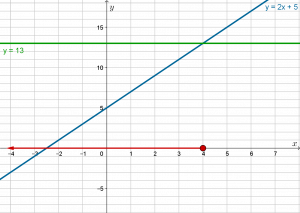# Multiply or Divide by Negative

Example 4 An inequality can be solved in the same way as an equation, however we must take care when multiplying by a negative number.

First, let’s divide by a positive number. SolveAny value ofgreater thanwill satisfyis greater than.

Example 5 Now let’s see what happens when both sides are negative. SolveUnlike the equationsand; the inequalities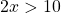anddo not yield the same solution.

Let’s solve:The inequalityhas solution.

The inequalityhas solution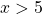.

When we multiply or divide by a negative number, the inequality sign is reversed.

Let’s solve again:Compare these results graphically: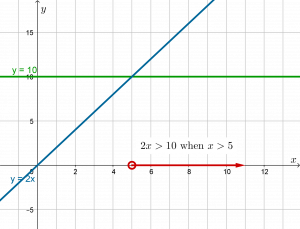One way to solve a quadratic inequality is to sketch a graph of the parabola, paying particular attention to the-intercepts (critical values).

First, rearrange the inequality to the formor. Then solve the corresponding equation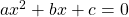. The solution interval(s) will one of:

1. a single interval between the two roots,2. two intervals, left ofand right of:or3. no solutions. The parabola does not cut the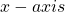and no values ofsatisfy the original equation;
4.. The parabola does not cut theand all values ofsatisfy the original equation.

Sketch a graph of the related parabola to determine the correct solution set.

## Example 6

SolveLet’s factor to find-intercepts:Theintercepts satisfyor, that is,and. Plot these two points and sketch an ‘upwards’ parabola.

Looking at the graphwe see that thecoordinate is less than zero on the interval highlighted in red.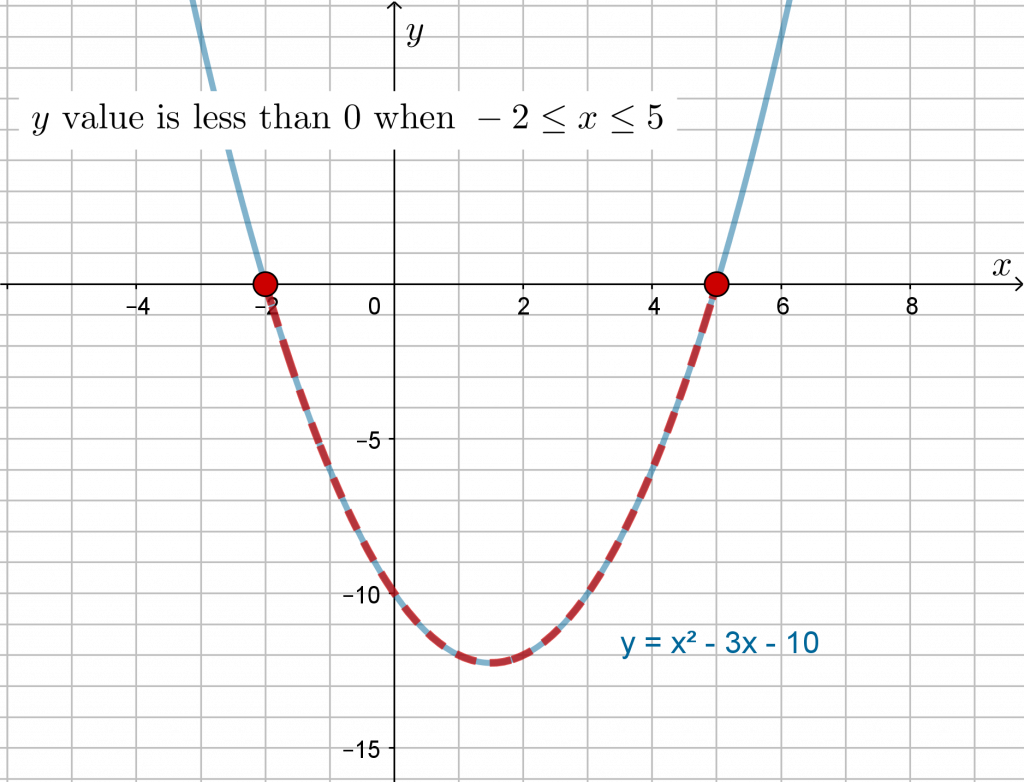Example 7

SolveWe are given the vertex form of this parabola. Let’s plot the key features:

Vertex has coordinates-intercept has coordinates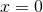,.

To calculate-intercepts, set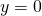and solve. It is possible to solve this directly when the parabola is given in vertex form, as follows: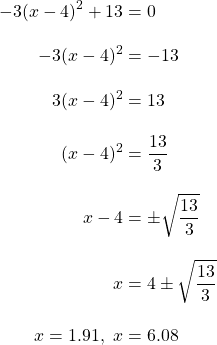Plot these five points and draw the parabola.We can now read that the values ofwhenor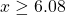.

Example 8

SolveLet’s begin by rearranging so that one side is zero:If it is a good day, the quadratic expression will factor:Sketch the parabola, paying attention to the-intercepts to see the solution interval  is.